# Taylor series

## Definition

Suppose$f$ is a function that is infinitely differentiable at a point$x_0$ in its domain. The Taylor series of$f$ about$x_0$ is the power series given as follows: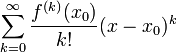$\sum_{k=0}^\infty \frac{f^{(k)}(x_0)}{k!} (x - x_0)^k$

Here's a version with the first few terms written explicitly: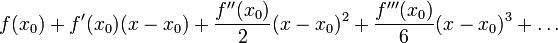$f(x_0) + f'(x_0)(x - x_0) + \frac{f''(x_0)}{2}(x - x_0)^2 + \frac{f'''(x_0)}{6}(x - x_0)^3 + \dots$

In the special case of the above definition where$x_0 = 0$ (and in particular$f$ is infinitely differentiable at 0), the Taylor series is as follows: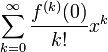$\sum_{k=0}^\infty \frac{f^{(k)}(0)}{k!} x^k$

Here's a version with the first few terms written explicitly:$f(0) + f'(0)x + \frac{f''(0)}{2}x^2 + \frac{f'''(0)}{6}x^3 + \dots +$

## Well defined on germs of a functions

The Taylor series operator about a point$x_0$ can be thought of as a mapping:

(Germs of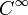$C^\infty$-functions defined about$x_0$)$\to$ (Formal power series centered at$x_0$)

In fact, this mapping is a$\R$-algebra homomorphism that commutes with the differential structure.

Here, two functions$f$ and$g$ are said to have the same germ about a point$x_0$ if there is an open interval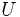$U$ containing$x_0$ such that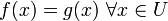$f(x) = g(x) \ \forall x \in U$.

## Relation with Taylor polynomials

The Taylor series can be viewed as a limit of Taylor polynomials. The$n^{th}$ Taylor polynomial for a function$f$ at a point$x_0$ in the domain is the truncation of the Taylor series to powers up to the$n^{th}$ power. If we denote the polynomial by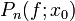$P_n(f;x_0)$, it is given as:$P_n(f;x_0) = x \mapsto \sum_{k=0}^n \frac{f^{(k)}(x_0)}{k!}(x - x_0)^k$

Note that this is a polynomial of degree at most$n$. The degree is exactly$n$ if and only if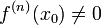$f^{(n)}(x_0) \ne 0$.

Whether or not the Taylor series of a function converges to the function is determined by whether or not the sequence of Taylor polynomials of the function converges to the function.

## Computation of Taylor series

To calculate the Taylor series of a function$f$ at a point$x_0$, we use the following procedure:

• Compute formal expressions for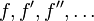$f,f',f'',\dots$, i.e.,$f$ and all its derivatives, at a generic point.
• Evaluate all these at$x_0$.
• Plug the result into the Taylor series formula.

### Example of exponential function

For further information, refer: Exponential function#Taylor series

Consider the exponential function:$f = \exp$

i.e.,$f(x) = e^x$.

We want to compute the Taylor series of this function at 0.

Applying the procedure above, we get:

• Formal expressions for$f,f',f'',\dots$: These are$\exp,\exp,\exp,\dots$. The sequence is a constant sequence of functions with all its members equal to$\exp$.
• Evaluate at 0: Since$\exp(0) = 1$, we get$1,1,1,\dots$. The sequence is a constant sequence with value 1 in all places.
• Plug the result into the Taylor series formula: We get:

Taylor series for$\exp(x)$ is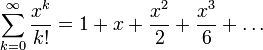$\sum_{k=0}^\infty \frac{x^k}{k!} = 1 + x + \frac{x^2}{2} + \frac{x^3}{6} + \dots$

Note that it is also true that the Taylor series for the exponential function converges to the exponential function everywhere; this is because the function is globally analytic. However, this fact is not a priori obvious, and we are not asserting it as part of the computation of the Taylor series.

### Example of cosine function

For further information, refer: Cosine function#Taylor series

Consider the cosine function: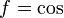$f = \cos$

We want to compute the Taylor series of this function at 0.

Applying the procedure above, we get:

• Formal expressions for$f,f',f'',\dots$: These are$\cos, -\sin, -\cos, \sin, \cos, -\sin, -\cos, \sin, \dots$. The sequence is periodic with period 4.
• Evaluate at 0: Since$\cos 0 = 1, \sin 0 = 0$, the values are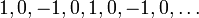$1,0,-1,0,1,0,-1,0,\dots$. The sequence is periodic with period 4.
• Plug the result into the Taylor series formula: We get:

Taylor series for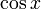$\cos x$ is$1 - \frac{x^2}{2!} + \frac{x^4}{4!} - \frac{x^6}{6!} + \dots$ which can be rewritten compactly as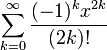$\sum_{k=0}^\infty \frac{(-1)^kx^{2k}}{(2k)!}$. Note that the$k$ here is half the exponent on$x$, so this is a little different from the usual way of writing Taylor series.

Note that it is also true that the Taylor series for the cosine function converges to the cosine function everywhere; this is because the function is globally analytic. However, this fact is not a priori obvious, and we are not asserting it as part of the computation of the Taylor series.

### Example of sine function

For further information, refer: Sine function#Computation of Taylor series

Consider the sine function: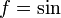$f = \sin$

We want to compute the Taylor series of this function at 0.

Applying the procedure above, we get:

• Formal expressions for$f,f',f'',\dots$: These are$\sin, \cos, -\sin, -\cos, \sin, \cos, -\sin, -\cos, \dots$. The sequence is periodic with period 4.
• Evaluate at 0: Since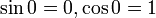$\sin 0 = 0, \cos 0 = 1$, the values are$0,1,0,-1,0,1,0,-1,\dots$. The sequence is periodic with period 4.
• Plug the result into the Taylor series formula: We get:

Taylor series for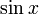$\sin x$ is$x - \frac{x^3}{3!} + \frac{x^5}{5!} - \frac{x^7}{7!} + \dots$ which can be rewritten compactly as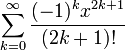$\sum_{k=0}^\infty \frac{(-1)^kx^{2k + 1}}{(2k + 1)!}$. Note that the$k$ here is roughly half the exponent on$x$, so this is a little different from the usual way of writing Taylor series.

Note that it is also true that the Taylor series for the sine function converges to the sine function everywhere; this is because the function is globally analytic. However, this fact is not a priori obvious, and we are not asserting it as part of the computation of the Taylor series.

## Facts

### Preservation of structure

Together, the first three facts show that the Taylor series operator is a homomorphism of$\R$-algebras that commutes with the differential structure. The fourth fact show that it preserves an additional structure:

### Convergence to the original function

• Composite of Taylor series operator and power series summation operator is identity map: What this essentially says is that if a power series centered at$x_0$ converges to$f$ on an open interval centered at$x_0$, then the power series must equal the Taylor series of$f$.
• Composite of power series summation operator and Taylor series operator is not identity map: It is possible to have an everywhere infinitely differentiable function$f$ and a point$x_0$ in the domain such that the sum of the Taylor series of$f$ at$x_0$ is not equal to$f$ on any interval of positive radius centered at$x_0$. In fact, we can arrange our example so that the power series sum agrees with$f$ only at$x_0$. Note that if this happens, then there cannot be any other power series centered at$x_0$ that converges to$f$ on a positive radius of convergence.

A function whose Taylor series at a point converges to the function in an open interval centered at the point is termed a locally analytic function at the point. If the Taylor series converges to the function everywhere, the function is termed a globally analytic function. We have that locally analytic not implies globally analytic.